# Test: Combinational Circuit- 1

## 10 Questions MCQ Test GATE Computer Science Engineering(CSE) 2023 Mock Test Series | Test: Combinational Circuit- 1

Description
Attempt Test: Combinational Circuit- 1 | 10 questions in 30 minutes | Mock test for Computer Science Engineering (CSE) preparation | Free important questions MCQ to study GATE Computer Science Engineering(CSE) 2023 Mock Test Series for Computer Science Engineering (CSE) Exam | Download free PDF with solutions
QUESTION: 1

### The term Product-of-sum in Boolean algebra means

Solution:

The Product of Sum (POS) expression comes from the fact that two or more sums (OR's) are added (AND'ed) together. That is the outputs from two or more OR gates are connected to the input of an AND gate so that they are effectively AND'ed together to create the final (OR AND) output.

QUESTION: 2

### Which expression is computed by the following NAND-gate circuit diagram: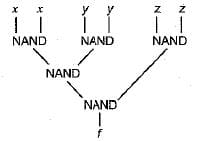Solution: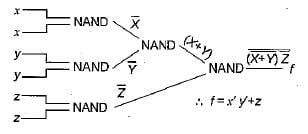QUESTION: 3

### What is the Boolean expression for the following circuit: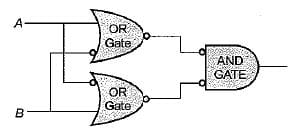Solution: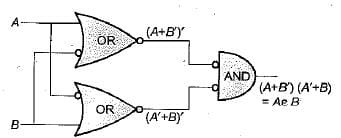QUESTION: 4

A multiplexer is also known as

Solution:

In electronics, a multiplexer (or mux; spelled sometimes as multiplexor), also known as a data selector, is a device that selects between several analog or digital input signals and forwards the selected input to a single output line.

QUESTION: 5

The quantity 837 in excess-3 BCD code would be represented as

Solution:

The quantity 837 is first converted to BCD representation then it is incremented by 3 bit by bit.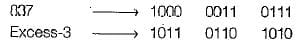QUESTION: 6

Solution:

A half adder is also known as XOR gate because XOR is applied to both inputs to produce sum.

QUESTION: 7

A comparison between serial and parallel adder reveals that serial order

Solution:

Comparison between serial and parallel adder reveals that serial adder is serial adder is a sequential circuit. A parallel adder is a combinational circuit. In serial adder, propagation delay is less. In parallel adder, propagation delay is present from input carry to output carry.

QUESTION: 8

How many full adders are needed to construct an m-bit parallel adder ;

Solution:

We can construct a m-bit parallel adder by,
iii) (2m - 1 ) full adders and (n - 1) OR GATES

QUESTION: 9

Identify the logic function performed by the circuit shown in the given figure: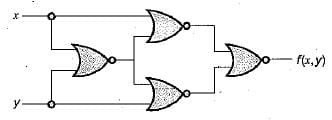Solution: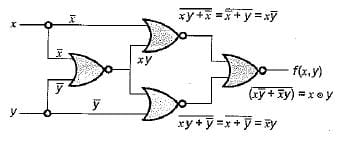Therefore the above circuit performed exclusive NOR gate.

QUESTION: 10

The logic expression for the output of the circuit shown in the figure below is: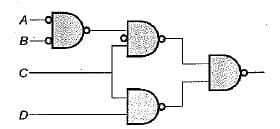Solution: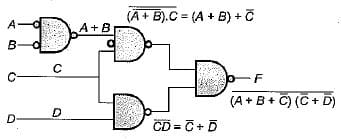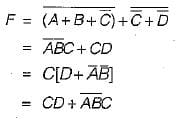Use Code STAYHOME200 and get INR 200 additional OFF Use Coupon Code